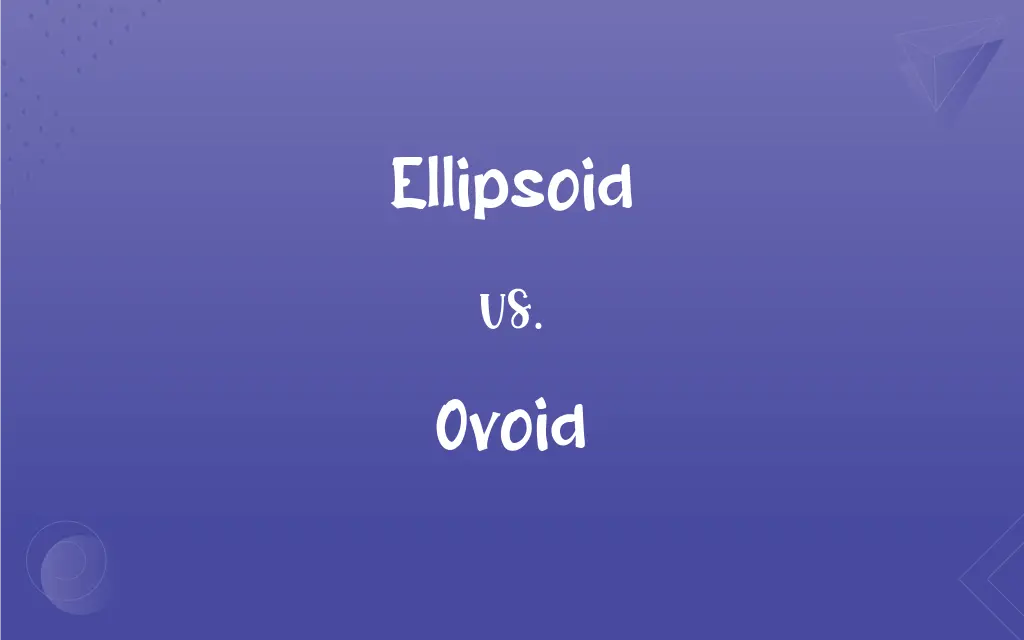# Ellipsoid vs. Ovoid: What's the Difference?Edited by Huma Saeed || By Sumera Saeed || Published on November 20, 2023
An ellipsoid is a three-dimensional shape with elliptical cross-sections, while an ovoid is a broadly egg-shaped object, more irregular than an ellipsoid.## Key Differences

An ellipsoid is a geometric figure, a three-dimensional version of an ellipse. It has symmetrical, elliptical cross-sections and can have three distinct axes of symmetry.
Ovoid, on the other hand, refers to shapes that are broadly egg-like. It suggests a more organic, less mathematically defined form, often with one end larger than the other.
The ellipsoid is a precisely defined geometric term. Its shape can be mathematically described using equations, often used in physics and engineering.
In contrast, ovoid is a more general descriptor. It's used in various contexts, from biology to art, to describe shapes that are egg-like but not necessarily symmetrical or geometrically precise.
While both describe three-dimensional shapes, ellipsoids are mathematically exact, while ovoids are more loosely defined, capturing the essence of egg-like forms.

## Comparison Chart

### Definition

3D shape, elliptical forms

### Symmetry

Symmetrical, defined axes
Generally asymmetrical

### Usage

Mathematics, physics
Biology, general description

### Precision

Mathematically precise
More organic, less precise

### Shape Regularity

Regular, geometric
Irregular, organic

## Ellipsoid and Ovoid Definitions

#### Ellipsoid

3D elliptical shape.
The earth is an oblate ellipsoid.

#### Ovoid

Loosely egg-shaped.
The vase had an ovoid appearance.

#### Ellipsoid

Geometry term.
We studied ellipsoids in math class.

#### Ovoid

She painted an ovoid pattern.

#### Ellipsoid

Symmetrical 3D figure.
He modeled an ellipsoid in his project.

#### Ovoid

Egg-shaped.
The ovoid sculpture stood in the garden.

#### Ellipsoid

Elliptical cross-sections.
The lamp’s ellipsoid design was unique.

#### Ovoid

Organic, asymmetrical shape.
The ovoid rock was intriguing.

#### Ellipsoid

Three axes of symmetry.

#### Ovoid

Not mathematically precise.
The artist preferred ovoid forms.

#### Ellipsoid

A geometric surface, all of whose plane sections are either ellipses or circles.

#### Ovoid

Shaped like an egg
An ovoid fruit.
Ovoid spots.

#### Ellipsoid

A surface, all of whose cross sections are elliptic or circular (including the sphere), that generalises the ellipse and in Cartesian coordinates (x, y, z) is a quadric with equation x2/a2 + y2/b2 + z2/c2 = 1. Category:en:Surfaces

## FAQs

#### Are ellipsoids found in nature?

Rarely, most natural forms are more ovoid.

#### Can any egg-shaped object be ovoid?

Generally, if it resembles an egg.

#### Is an ellipsoid always symmetrical?

Yes, it has symmetrical axes.

#### Does ovoid imply irregularity?

It suggests organic, less precise shapes.

#### Are all ellipsoids 3D?

Yes, by definition.

#### Is ovoid a precise geometric term?

No, it's more descriptive than precise.

#### Can ovoid shapes be symmetrical?

Occasionally, but often they're not.

#### Can an ellipsoid have circular cross-sections?

Yes, in special cases like a sphere.

#### Is ellipsoid only a mathematical term?

Primarily, but used in other fields too.

#### Can ellipsoids be flattened?

Yes, like an oblate ellipsoid.

#### Does ellipsoid have a fixed formula?

Yes, its shape is mathematically defined.

#### Do ovoid shapes have uniform thickness?

Not necessarily; they can be variable.

#### Can ovoid be used in architecture?

Yes, for organic, egg-like designs.

#### Is ovoid used in biology?

Frequently, to describe cell shapes.

#### Are ovoid shapes common in nature?

Yes, many natural forms are ovoid.

#### Do artists use the term ovoid?

Yes, for egg-like forms in art.

#### How many axes does an ellipsoid have?

Usually three distinct axes.

#### Does ovoid imply a specific size?

No, it refers to shape, not size.

#### Are ellipsoids used in astronomy?

Yes, for celestial bodies' shapes.

#### Can ellipsoids be elongated?

Yes, like a prolate ellipsoid.